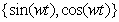Archive
Special IssuesVolume 4, Issue 2, April 2016, Page: 74-80
Implicit Exponentially Fitted RKNd Methods for Solving Oscillatory ODEs
Wenjuan Zhai, Department of Mathematics, Beijing Jiaotong University Haibin College, Cangzhou, P. R. China
Bingzhen Chen, Department of Applied Mathematics, Beijing Jiaotong University, Beijing, P. R. China
Received: Apr. 12, 2016;       Published: Apr. 13, 2016
Abstract
In this paper, we derive the implicit exponentially fitted RKNd methods for solving oscillatory ODEs. The new methods integrate exactly differential systems whose solutions can be expressed as linear combinations of functions from the set {exp(λt), exp(−λt)}, λ ∈ C, or equivalentlywhen λ = iω, ω ∈ R. Numerical experiments are accompanied to show the efficiency and competence of the implicit exponentially fitted RKNd methods compared with implicit RKNd methods.
Keywords
RKNd Method, Exponentially Fitted, Implicit, Stability, Efficiency, Oscillatory
Wenjuan Zhai, Bingzhen Chen, Implicit Exponentially Fitted RKNd Methods for Solving Oscillatory ODEs, Science Journal of Applied Mathematics and Statistics. Vol. 4, No. 2, 2016, pp. 74-80. doi: 10.11648/j.sjams.20160402.19
Reference

P. Albrecht, The extension of the theory of A-methods to RK methods. In: Strehmel, K. (Ed.), Numerical Treatment of Differential Equations, Proceedings of the 4th Seminar NUMDIFF-4, Tuebner-Texte Zur Mathematik. Tuebner, Leipzig 1987, 8-18.

P. Albrecht, A new theoretical approach to Runge Kutta methods, SIAM J. Numerical Anal., 1987, 24: 391-406.

Blanes S. Explicit symplectic RKN methods for perturbed non-autonomous oscillators: Splitting, extended and exponentially fitting methods, Computer Physics Communications, 2015, 193: 10-18.

B. Zh. Chen and X. You. RKNd methods for solving initial value problems, Numer. Math. Sinica., 2010, 32(4): 399-412. (in Chinese).

D’Ambrosio R, Paternoster B, Santomauro G. Revised exponentially fitted Runge–Kutta–Nyström methods, Applied Mathematics Letters, 2014, 30(30): 56-60.

E. Hairer, S P. Nφrsett and G. Wanner, Solving ordinary differential equations I, Nonstiff problems [M]. Berlin: Springer-Verlag 1993.

J C. Butcher, The Numerical Analysis of Ordinary Differential Equations [M]. Wiley: Chichester UK 1987.

W. Gautschi, Numerical integration of ordinary differential equations based on trigonometric polynomials, Numer. Math., 1961, 3: 381-397.

T. Lyche, Chebyshevian multistep methods for ordinary differential equations, Numer. Math., 1972, 19: 65-75.

B. Paternoster, Runge-Kutta(-Nyström) methods for ODEs with periodic solutions based on trigonometric polynomials, Appl. Numer. Math., 1998, 28: 401-412.

G. Vanden Berghe, H. De Meyer, M. Van Daele and T. Van Hecke, Exponentially fitted Runge-Kutta methods, J. Comp. Appl. Math., 2000, 125: 107-115.

J. M. Franco, An embedded pair of exponentially fitted explicit Runge-Kutta methods, J. Comput. Appl.Math., 2002, 149: 407-414.

J. M. Franco, Exponentially fitted explicit Runge-Kutta -Nyström methods, J. Comput. Appl. Math., 2004, 167: 1-19.

J. M. Franco, I. Gómez, Symplectic explicit methods of Runge–Kutta–Nyström type for solving perturbed oscillators. Journal of Computational & Applied Mathematics, 2014, 260: 482-493.

L. Gr. Ixaru and G. Vanden Berghe, Exponential Fitting, Kluwer Academic Publishers, Dordrecht, 2004.

T. Monovasilis, Z. Kalogiratou, T E. Simos, Construction of Exponentially Fitted Symplectic Runge–Kutta–Nyström Methods from Partitioned Runge–Kutta Methods. Mediterranean Journal of Mathematics, 2014, 1618(1): 1-15.

T. E. Simos and J. Vigo-Aguiar, Exponentially fitted symplectic integrator, Phys. Rev. E., 2003, 67: 1-7.

H. Van de Vyver, Runge-Kutta type methods for periodical initial value problems, PhD thesis, Catholic University of Leuven, 2006.

Hans Van de Vyver, A Runge-Kutta-Nyström pair for the numerical integration of perturbed oscillators, Comput. Phys. Commun., 2005, 167: 129-142.

R. Van Dooren, Stabilization of Cowell’s classical finite difference method for numerical integration, J. Comput. Phys., 1974, 16: 186-192.

W. J. Zhai, B. Zh. Chen, Exponentially Fitted RKNd Methods for Solving Oscillatory ODEs, Advances in Mathematics, 2013, 42(3): 393-403.

D. Zhao, Z. Wang and Y. Dai, Importance of the first-order derivative formula in the Obrechkoff methods, Comput. Phys. Commun., 2005, 167: 65-75.